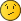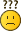# LDraw.org Discussion Forums

Full Version: Heightmap to brick converter?
You're currently viewing a stripped down version of our content. View the full version with proper formatting.
Pages: 1 2 3
Does anyone know of any heightmap to LDraw brick converters? I know MLCad can create fractal terrain, but I could have sworn someone had written a converter at some point. Thanks.
Thanks! But, there seems to be a limit on the "max size" a model can be. Not sure how to get around that.
(2015-08-14, 10:28)Michael Horvath Wrote: [ -> ]Does anyone know of any heightmap to LDraw brick converters? I know MLCad can create fractal terrain, but I could have sworn someone had written a converter at some point. Thanks.

Hello,

I am currently looking for the same.

Digging LDraw history (http://www.ldraw.org/community/history/), I found Jacob Sparre Anderson made a Fractal Landscape Editor way back in 1997.
I even found some code on Github referencing to it: https://github.com/sparre/LEGO-tools

I wrote a basic Excel sheet that can generate a `landscape` on a 16x16 baseplate using 1x1 plates and bricks with a maximum height of one brick.
So that is either 1 plate, 2 plates or 1 brick.

Not nearly what I am looking for or what Michael meant.

Does anyone have an idea or tool that can do this?
I am no programmer, but it seems the basics have been made, but unfortunately never worked out further.

Another idea would be using a mosaic generator of which there are quite a few of that can make LDraw files.
But then you have no height, but somehow the color could determine this.
Just some thoughts...
(2015-08-15, 5:21)Michael Horvath Wrote: [ -> ]Thanks! But, there seems to be a limit on the "max size" a model can be. Not sure how to get around that.
What area (in studs/baseplates) are you talking about?

Do you want to import a greyscale image that represents a height map?
(2018-06-18, 13:40)Gerald Lasser Wrote: [ -> ]
(2015-08-15, 5:21)Michael Horvath Wrote: [ -> ]Thanks! But, there seems to be a limit on the "max size" a model can be. Not sure how to get around that.
What area (in studs/baseplates) are you talking about?

Do you want to import a greyscale image that represents a height map?

My project is based on x and z coordinates of dots in a grid that have a certain height.
The source would be an excel file.
What I am looking for is a way to translate that, using 1x1 plates and bricks laying out a mosaic like "terrain".

What I can make now is this:
A dot at point x,z at height 1 translates to a 1x1 plate
A dot at height 2  translates to two stacked 1x1 plates
A dot at height 3 translates to a 1x1 brick

The next step I want to make is to be able to calculate the stack at any height.
So a dot at height 4 is a brick and one plate stacked
A dot at height 5 is a brick and two plates stacked
Height 6 is 2 bricks
Height 7 is 2 bricks and one plate
And so forth.

No idea so far how to do that.
Any thoughts.(2018-06-18, 20:14)Jaco van der Molen Wrote: [ -> ]No idea so far how to do that.
Any thoughts.If you can somehow generate a lua variable definition with excel.

Then you can do the actual generating of the model in LDCad using a macro. All you would need is the x/z values in an array.

For an 3x3 grid you would need something like this:

Code:
`local depthMap={ {0, 1, 2}, {4, 5, 6}, {7, 8, 9} }`

You use that in a macro run event like so:

Code:
```function renDepthGen()  local sf=ldc.subfile()  if not sf:isLinked() then    ldc.dialog.runMessage('No active session')    return  end    local sel=ldc.selection()  sel:remove()  local depthMap={    {0, 1, 2},    {4, 5, 6},    {7, 8, 9}  }    local h=genTools.tableArrayCount(depthMap)  local w=genTools.tableArrayCount(depthMap)    for x=1,w do    for z=1,h do      local v=depthMap[z][x]            local v1=v // 3      local v2=v % 3          local xx=(x-1)*20      local zz=(z-1)*20      local yy=0            for b=1,v1  do        yy=yy-24        sel:add(sf:addNewRef('3005.dat', 4, ldc.matrix(xx, yy, zz)))            end            for p=1,v2 do        yy=yy-8        sel:add(sf:addNewRef('3024.dat', 4, ldc.matrix(xx, yy, zz)))      end          end  end end```

Which would give you:
[attachment=3196]
(2018-06-18, 20:56)NRoland Melkert Wrote: [ -> ]
(2018-06-18, 20:14)Jaco van der Molen Wrote: [ -> ]No idea so far how to do that.
Any thoughts.If you can somehow generate a lua variable definition with excel.

Then you can do the actual generating of the model in LDCad using a macro. All you would need is the x/z values in an array.

For an 3x3 grid you would need something like this:

Code:
`local depthMap={ {0, 1, 2}, {4, 5, 6}, {7, 8, 9} }`

You use that in a macro run event like so:

Code:
```function renDepthGen()  local sf=ldc.subfile()  if not sf:isLinked() then    ldc.dialog.runMessage('No active session')    return  end    local sel=ldc.selection()  sel:remove()  local depthMap={    {0, 1, 2},    {4, 5, 6},    {7, 8, 9}  }    local h=genTools.tableArrayCount(depthMap)  local w=genTools.tableArrayCount(depthMap)    for x=1,w do    for z=1,h do      local v=depthMap[z][x]            local v1=v // 3      local v2=v % 3          local xx=(x-1)*20      local zz=(z-1)*20      local yy=0            for b=1,v1  do        yy=yy-24        sel:add(sf:addNewRef('3005.dat', 4, ldc.matrix(xx, yy, zz)))            end            for p=1,v2 do        yy=yy-8        sel:add(sf:addNewRef('3024.dat', 4, ldc.matrix(xx, yy, zz)))      end          end  end end```

Which would give you:

Ah! Ok, I see. That is exactly what I meant!
My excel source can make the depth map, I understand how it works.
For a 16 by 16 baseplate you have 16 arrays with each 16 values.
Now if you can incorporate the color of the column too, I am there.
(2018-06-18, 21:10)Jaco van der Molen Wrote: [ -> ]Ah! Ok, I see. That is exactly what I meant!
My excel source can make the depth map, I understand how it works.
For a 16 by 16 baseplate you have 16 arrays with each 16 values.
Now if you can incorporate the color of the column too, I am there.
You could choose between green and blue (instead of the constant red) depending on the height of 'yy' to mimic above/below sea level. Or do you want the whole column to vary in color depending on an excel variable?

edit, like so:

replcace the '4' in the addNewRef lines with
Code:
`genTools.IF(yy<seaLevel, 2, 1)`

Code:
`local seaLevel=-24`
above the main w/h loop.

ps: as you'll probably notice soon the script needs
Code:
`local genTools=require('genTools')`
at the top of the .lua file.
OK, got it to work smoothly. Very powerful.
Now for the color.

Got it.

Code:
```local colorMap={   { 1,  2,  3,  4 },   { 5,  6,  7,  8 },   { 9, 10, 11, 12 }  }```

and

Code:
`local c=colorMap[z][x]`

(right after local v=depthMap[z][x]

changed the hard coded color in this line to variable c:

Code:
`sel:add(sf:addNewRef('3005.dat', c, ldc.matrix(xx, yy, zz)))     `

I tried to implement it, but no succes.
Would that work?
Pages: 1 2 3